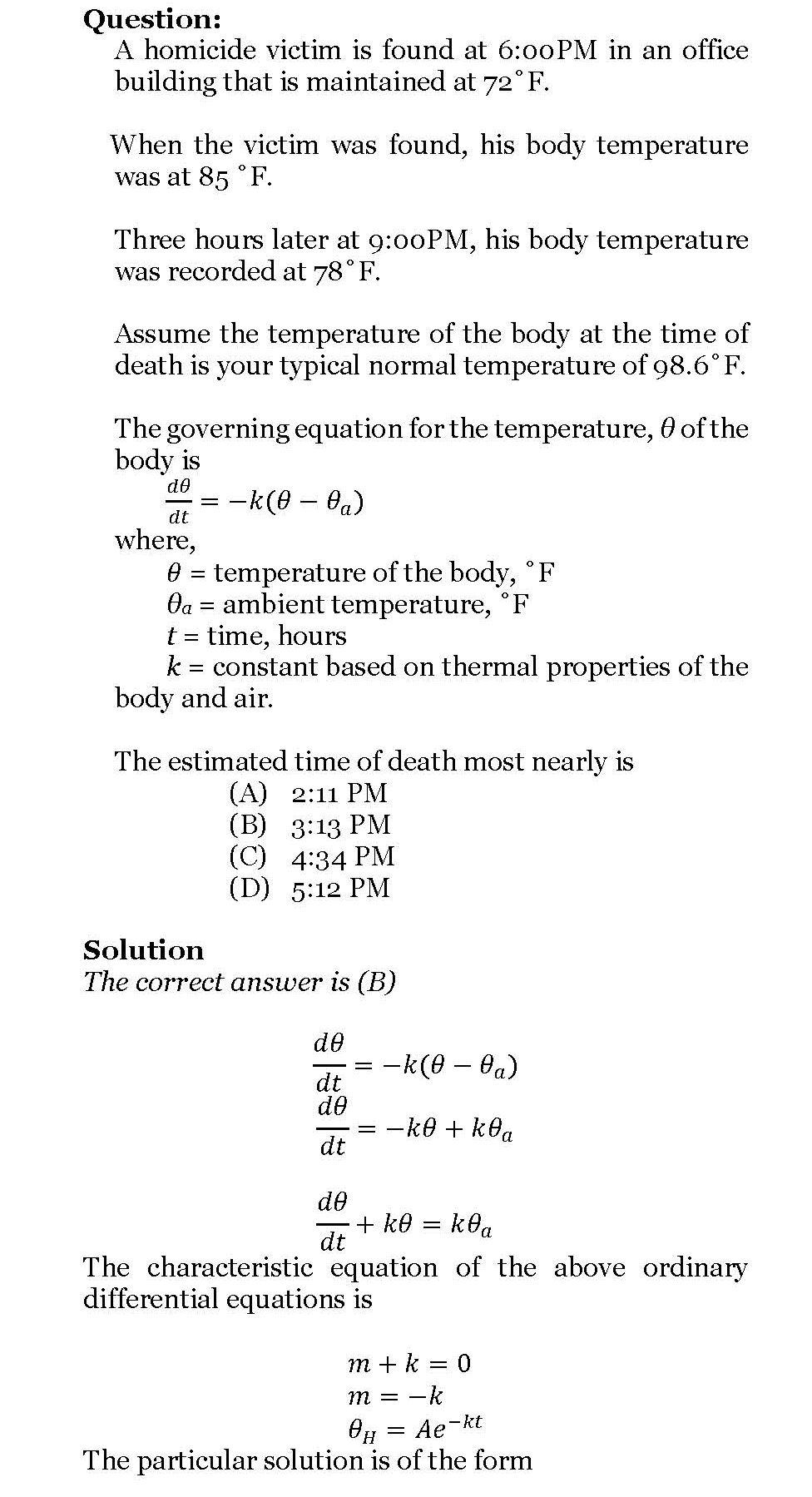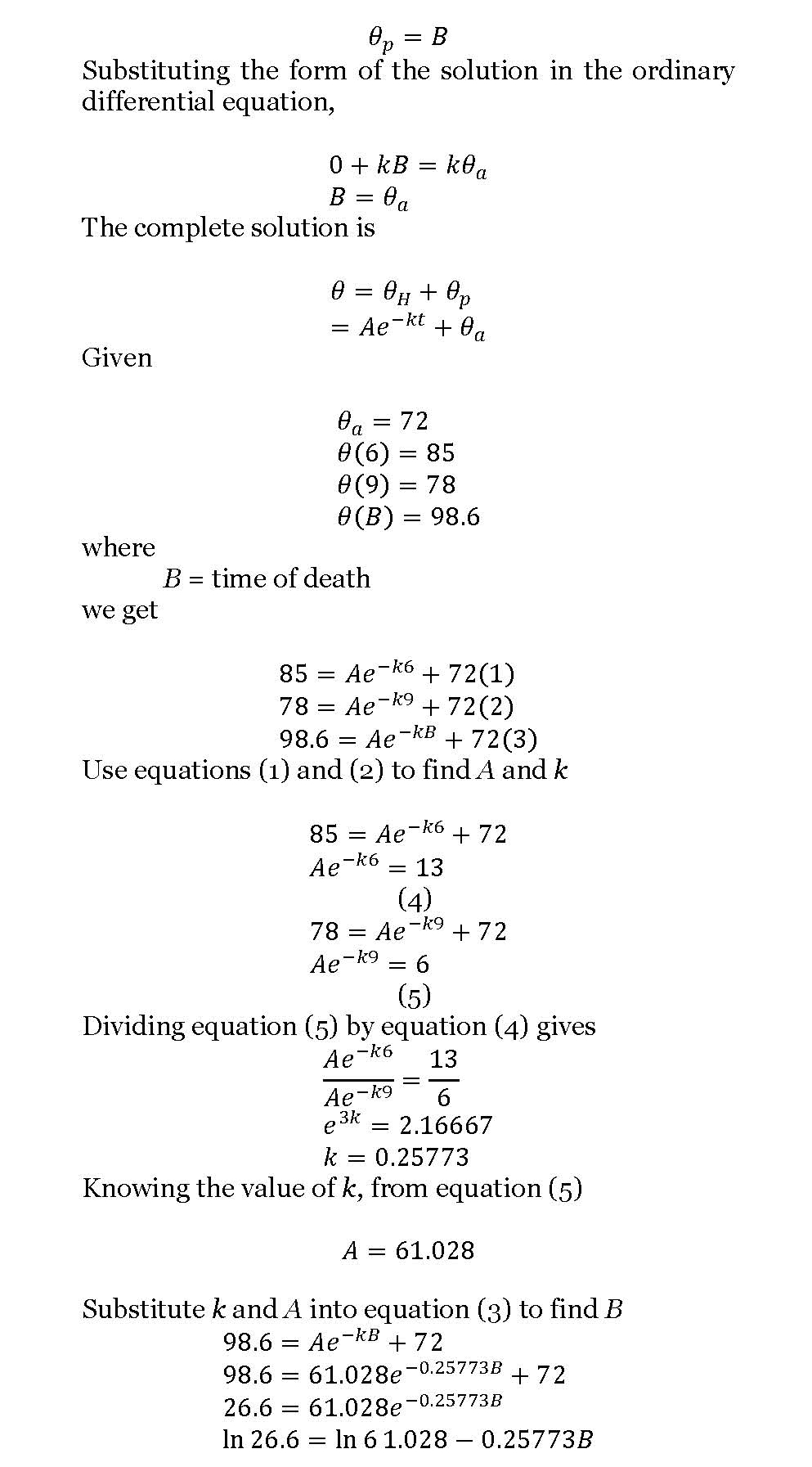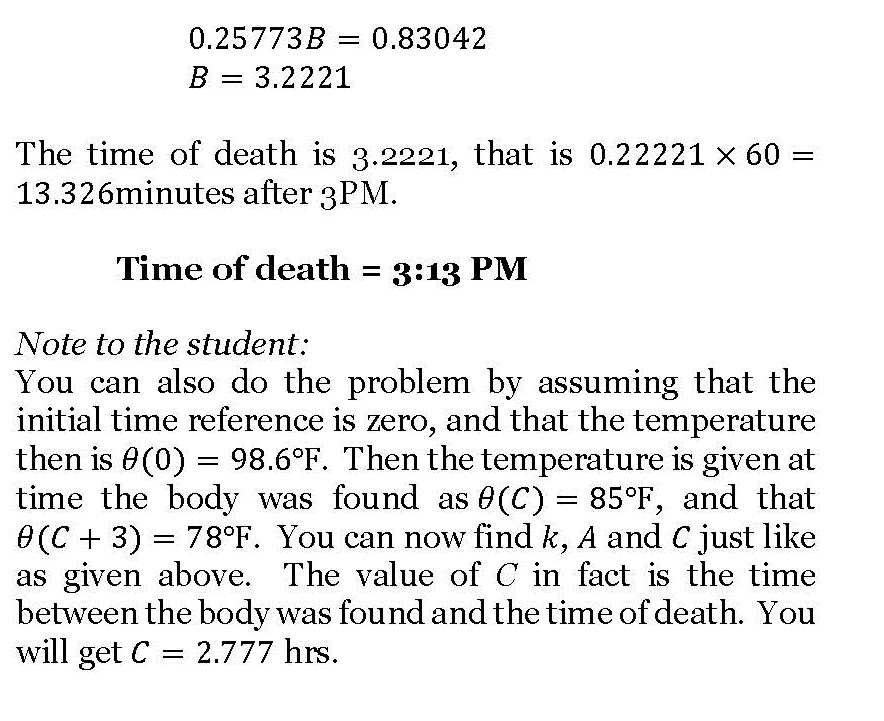# Time of death – a classic ODE problem

One of the classical applied problems in ordinary differential equations is that of finding the time of death of a homicide victim.

The estimation of time of death is generally based on the temperature of the body at two times – 1) when the victim is found and 2) then a few hours later. Assuming the ambient temperature stays the same and the body is treated as a lumped system, one can use a simple linear ODE to solve the problem.

It is somewhat an inverse problem as we are trying to find the value of the independent variable – the time of death. Here is a solved problem (a pdf version also available)._____________________________________________________

This post is brought to you by Holistic Numerical Methods: Numerical Methods for the STEM undergraduate at http://nm.mathforcollege.com

## 0 thoughts on “Time of death – a classic ODE problem”

1.Denaya says:

Dear Sir,

According to what I’ve read on eqworld.ipmnet.ru site at http://eqworld.ipmnet.ru/forum/viewtopic.php?f=2&t=34&st=0&sk=t&amp;
on the topic of Mathematical Equations posted by Janesh, the linear first order DE of homicide victim model dӨ/dt + kӨ = kӨa corresponds to zero (n=0) order of Bernoulli DE. On her post, Janesh introduced a so-called Rohedi’s formula for Bernoulli DE, maybe the formula can be used to verify your exact solution. Let we read together the detail explanation from Janesh.

%%%%%%%%%%%%%%%%%%%%%%
Rohedi’s Formula For BDE of constant coefficients
Post by janesh on 03 Nov 2008, 21:27
Dear Sir,

I am so interested to Rohedi’s Formula for BDE of constant coefficients as you introduced in your SMT’s paper on http://rohedi.com/content/view/25/26/:

dy/dx + p*y = Q*y^n, for n is not equal 1

that is of form :

y(x) = [ {y0^(1-n) – Q/p}*exp{-(1-n)p(x-x0)} + Q/p ]^{1/(1-n)}

Can you give us some applications of the Rohedi’s formula?

Thanks Mr.Rohedi,
Janesh.
%%%%%%%%%%%%%%%%%%%%

Now, I try to verify your result using rohedi’s formula, where for the following DE

dӨ/dt + kӨ = kӨa , (1)

I have several parameters x=t, y=Ө, p=k, Q=kӨa, and n=0. Hence, the general solution of eq.(1) is in the form :

Ө(t) = {Ө0 – Q/p}*exp{-p(t-t0)} + Q/p

or

Ө(t) = {Ө0 – Өa}*exp{-p(t-t0)} + Өa , (2)

For k=0.25773, and Өa=72, then eq.(2) becomes

Ө(t) = ( Ө0 – 72)*exp{-0.25773(t-t0)} + 72 , (3)

Next, inserting your initial values t0=B, Ө0=98.6 into eq.(3), then the exact solution of homicide victim is of form:

Ө(t) = ( 98.6 – 72)*exp{-0.25773(t-3.2221)} + 72, (4)

Verify of eq(4) can be performed by inserting t=6 into eq(4), that gives Ө=85.

Okay Sir, your calculation is right, and I am very glad now.

Thanks Sir.

Astrid Denaya Lesa.

2.Barbara says:

Thx Denaya Lesa.

3.Barbara says:

Thx Denaya Lesa.

4.Denaya Lesa says:

Sorry, Denaya has just known that there is nice comment for me. But, I think Barbara also would thank to Professor Autar Kaw who has accepted me in posting Rohedi’s formula of my father here. Thx.
5.Denaya Lesa says: